Courses

# RD Sharma Solutions (Part - 1) - Ex - 8.2, Linear Equations in One Variable, Class 7, Math Class 7 Notes | EduRev

## RD Sharma Solutions for Class 7 Mathematics

Created by: Abhishek Kapoor

## Class 7 : RD Sharma Solutions (Part - 1) - Ex - 8.2, Linear Equations in One Variable, Class 7, Math Class 7 Notes | EduRev

The document RD Sharma Solutions (Part - 1) - Ex - 8.2, Linear Equations in One Variable, Class 7, Math Class 7 Notes | EduRev is a part of the Class 7 Course RD Sharma Solutions for Class 7 Mathematics.
All you need of Class 7 at this link: Class 7

#### Question 1:

x − 3 = 5

x − 3 = 5
Adding 3 to both sides, we get
⇒ x − 3 + 3 = 5 + 3
⇒ x = 8
Verification:
Substituting x= 8 in LHS, we get
LHS = x − 3 and RHS = 5
LHS = 8 − 3 = 5 and RHS = 5
LHS = RHS
Hence, verified.

Question 2:

x +9  = 13

x + 9 = 13
Subtracting 9 from both sides, we get
=> x + 9 − 9 = 13 − 9
=> x = 4
Verification:
Substituting x = 4 on LHS, we get
LHS = 4 + 9 = 13 = RHS
LHS = RHS
Hence, verified.

#### Question 3: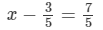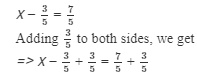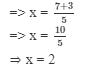Verification:
Substituting x = 2 in LHS, we get

LHS =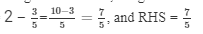LHS = RHS
Hence, verified.

#### Question 4:

3x = 0

3x = 0
Dividing both sides by 3, we get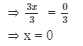Verification:

Substituting x = 0 in LHS = 3x, we get
LHS = 3 ×× 0 = 0 and RHS = 0
LHS = RHS
Hence, verified.

#### Question 5:

Solve each of the following equations and check your answes: x/2=0

x/2=0

Multiplying both sides by 2, we get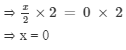Verification:

Substituting x= 0 in LHS, we get
LHS =0/2= 0 and RHS = 0
LHS = 0 and RHS = 0
LHS = RHS
Hence, verified.

#### Question 6: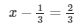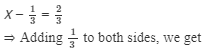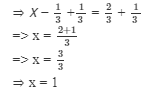Verification:

Substituting x= 1 in LHS, we get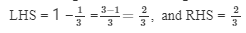LHS = RHS
Hence, verified.

#### Question 7: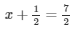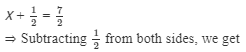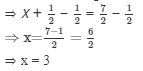Verification:

Substituting x = 3 in LHS, we get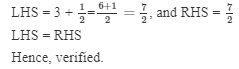#### Question 8:

10 − y = 6

10 − y = 6
Subtracting 10 from both sides, we get
⇒ 10 − y − 10 = 6 − 10
⇒ −y = −4.
⇒ Multiplying both sides by −1, we get
⇒ −y ×−1 = −4 ×−1
⇒ y = 4
Verification:
Substituting y = 4 in LHS, we get
LHS = 10 − y = 10−4 = 6 and RHS = 6
LHS = RHS
Hence, verified.

#### Question 9:

7 + 4y = −5

7 + 4y = −5
Subtracting 7 from both sides, we get
⇒ 7 + 4y − 7 = −5 − 7
⇒  4y  = −12
Dividing both sides by 4, we get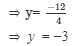Verification :
Substituting y = −3 in LHS, we get
LHS = 7 + 4y = 7  + 4(−3) = 7 − 12 = −5, and RHS = −5
LHS = RHS
Hence, verified.

#### Question 10: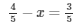Subtracting 4/5 from both sides, we get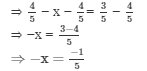Multiplying both sides by -1, we ge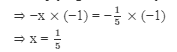Verification :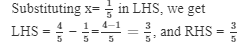LHS = RHS
Hence, verified.

#### Question 11: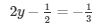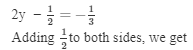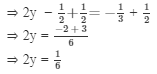Dividing both sides by 2, we get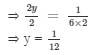Verification :

Substituting y = 1/12 in LHS, we get

LHS =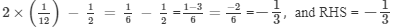LHS = RHS
Hence, verified.

Question 12: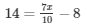Adding 8 to both sides, we get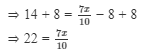Multiplying both sides by 10, we get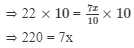Dividing both sides by 7, we get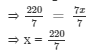Verification :

Substituting x = 220/7 in RHS, we get

LHS = 14, and RHS =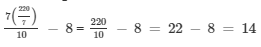LHS = RHS
Hence, verified.

Offer running on EduRev: Apply code STAYHOME200 to get INR 200 off on our premium plan EduRev Infinity!

97 docs

,

,

,

,

,

,

,

,

,

,

,

,

,

,

,

,

,

,

,

,

,

,

,

,

,

,

,

,

,

,

;